# Analytic Solution for Subharmonic Oscillation

2017.04.27

・Subharmonic oscillations occur when the duty cycle is above 50%.

・Although not directly related to transfer functions, it is important to understand this analytic solution for subharmonic oscillation.

In a step-down converter with a fixed frequency and with peak current control, the inductor current flows continuously, and it is well known that if the duty cycle is 50% or higher, subharmonic oscillations occur. In nearly all recent ICs for use in power supplies, a slope compensation circuit is provided to address this issue.

This time, we stray somewhat from derivation of transfer functions for DC-DC converters to explain an analytic solution for subharmonic oscillation, an understanding of which is important.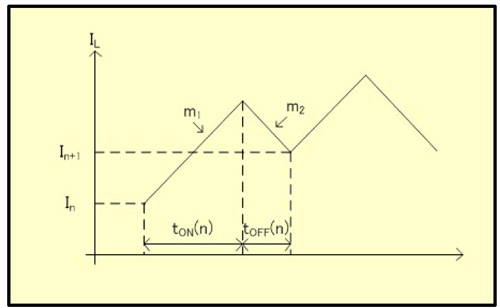Fig.10

The coil (inductor) current waveform in a current-mode step-down converter is similar to that in Fig. 10. Here the current value at a given time is In, and the current value one period later is In+1.

Further, the turn-on time is tON(n), the turn-off time is tOFF(n), the slope of the coil current while turned on is m1, and the slope of the coil current while turned off is m2.

These can be used to express In+1 in terms of In as in equation 3-15 below.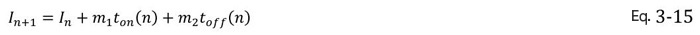Here, m1 and m2 can be expressed by equations 3-16 and 3-17 respectively.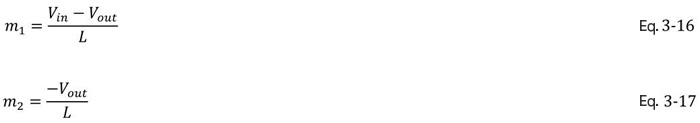Fig. 11 below shows a PWM input waveform corresponding to Fig. 10. If the current sense gain is RS, then immediately after turning on the PWM input is RSIn.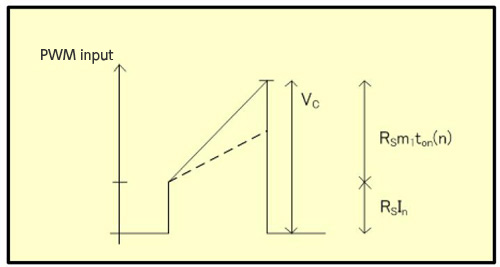Fig.11

Further, the current that has increased during tON becomes m1tON(n), and so the PWM input peak voltage VC is as expressed by equation 3-18.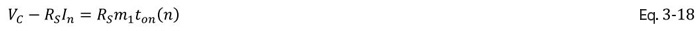Also, ｔOFF(n) in equation 3-15 can be rewritten as in equation 3-19 below. Here T is the period.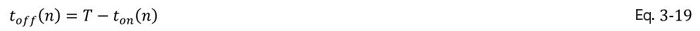From equation 3-18, tON(n) is found, and upon substitution into equation 3-15 and calculating, equation 3-20 is obtained.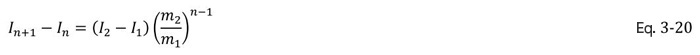｛In+1-In} is a geometric progression, and so the condition for not causing subharmonic oscillation is that this geometric progression converges to 0 as n → ∞. In other words, the condition is expressed by equation 3-21 below.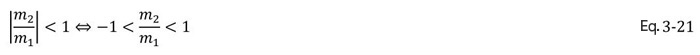Substituting equations 3-16 and 3-17 into 3-21, we obtain the following conditional formula 3-22.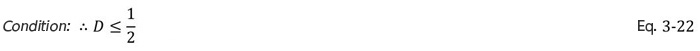Thus the condition for not causing subharmonic oscillation is that the duty cycle D be 1/2, that is, 50% or lower. Referring to our statement at the outset, then, we have derived the analytic solution indicating that at a duty cycle above 50%, subharmonic oscillations occur.